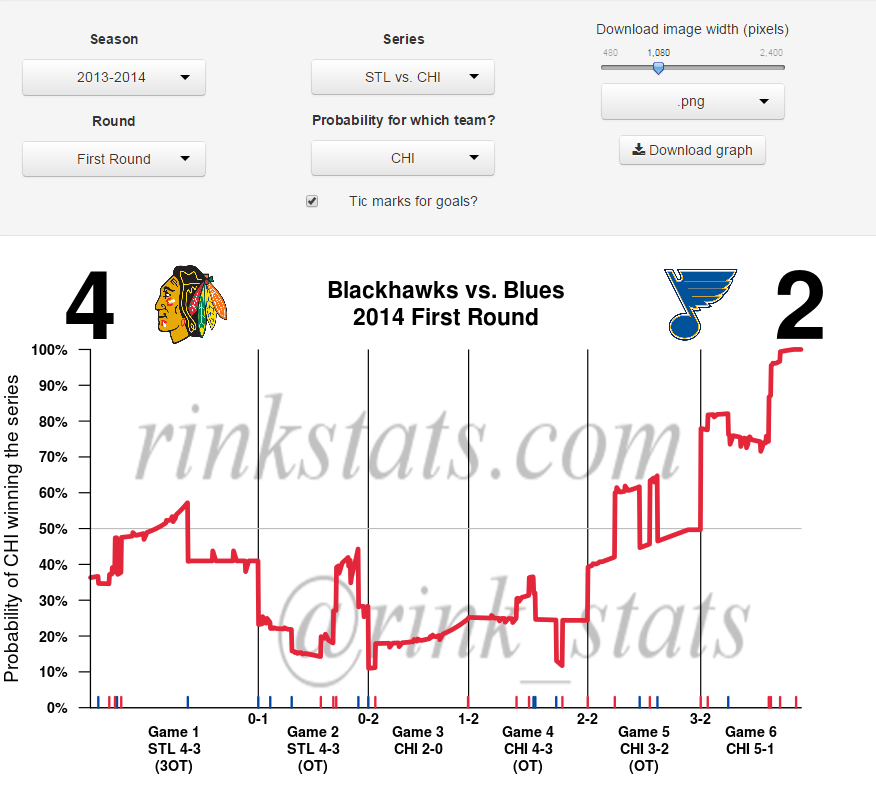# Playoff series win probabilities (plus a new app!)

## July 25, 2014

About a month ago I unveiled a web app that calculates in-game win probabilities for NHL games, based on the metric I developed here.

My next plan in the project was to turn to single game win probability metric into a statistic that gives us the probability that a team wins a playoff series at each second of the series. I’ve done this an turned it into another web app, which you can play with here.The app allows the user to choose any playoff series since 2008 and see the probability graph, as well as several summary statistics. The code is also written in a way so that when next year’s playoffs begin, the user will be able to get probabilities from those series.

### More about the statistics underlying the metric

The basic intuition behind the metric is that it combines my individual game win probability metric with basic data on the probability of winning a series, conditional on how many games the team has won and lost so far in the series.

To calculate the metric at all points of the playoff series, I first calculate the in-game probabilities from each individual game. I then use historical data (back to the 1970s expansion of the NHL to 12 teams) to get the probability of a team winning a playoff series, given how many games have been played, how many games that team had won, and how many home games that team had remaining. These provide the probability of winning the series at the start of each game.

I then use conditional probability to combine together the two pieces. The basic math is as follows.

Define C as the probability that a team wins the current game. Define W as the probability of winning the series if they win the current game. Define L as the probability of winning the series if they lose the current game. Then the probability of winning the series, at this exact moment is equal to CW + (1-C)(L). This formula allows me to rescale each game’s probabilities so that they all can be connected into a single line.

There’s two next steps on this project. The first is to do the final natural extension and create a statistic which gives the probability that a team wins the Stanley Cup at each point during the playoffs. The second idea that I’m working on is using the in-game statistics to create a player evaluation metric. I’ve got a few ideas on how to do that, so hopefully I’ll be able to roll that out in the next couple months.